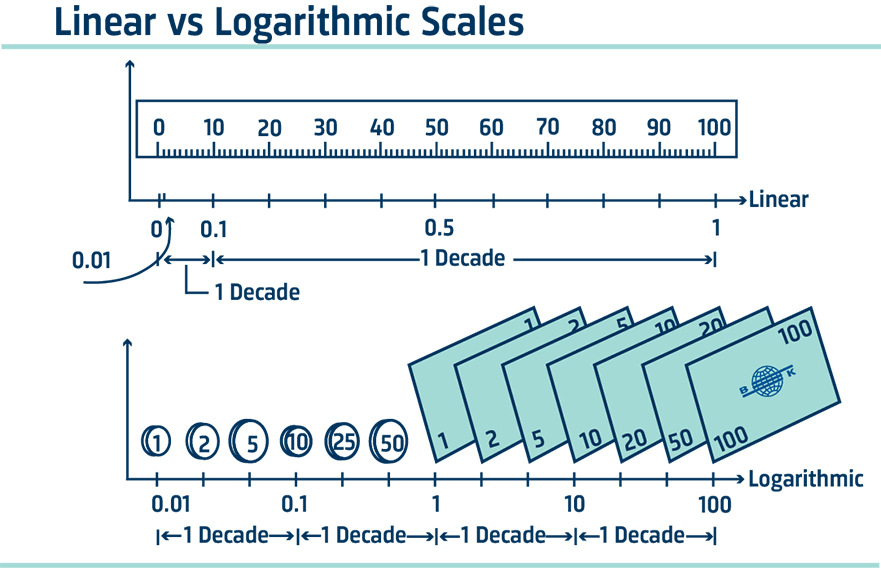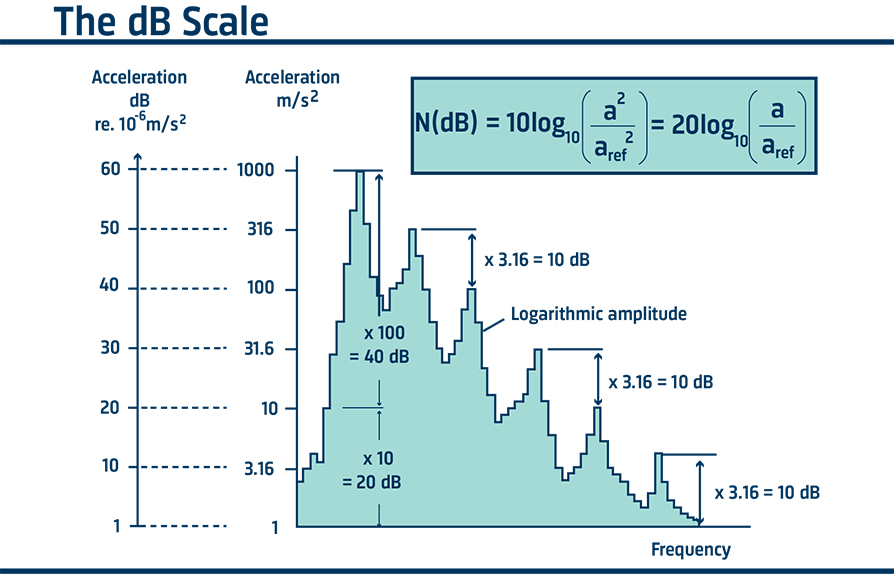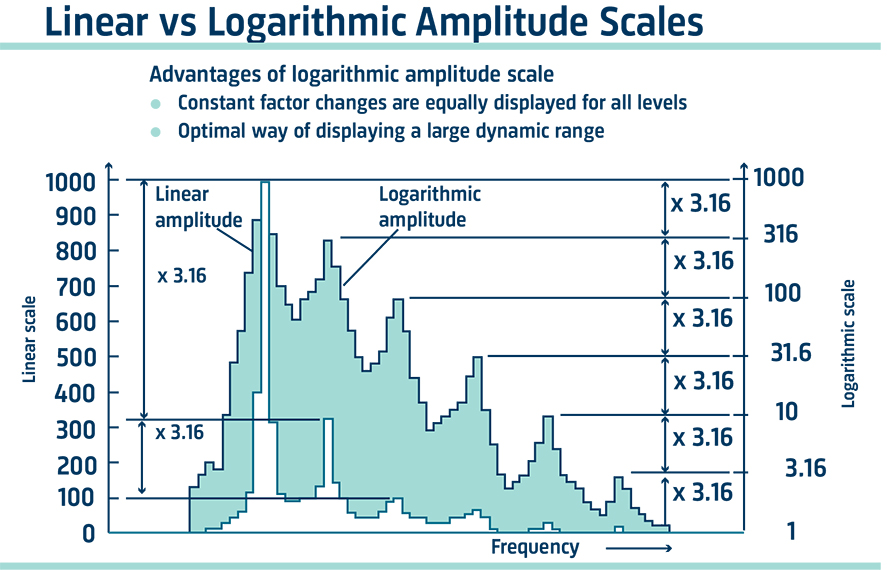# Calibrating an Accelerometer

Accelerometer calibration is part of the measuring vibration introduction which answers some of the basic questions asked by the newcomer to vibration measurement. It gives a brief explanation of the following: calibrators, force, and impedance measurements, and logarithmic scales and decibels.

GET THE FULL HANDBOOK
MEASURING VIBRATION
BY BRÜEL & KJÆR

Each Brüel & Kjær accelerometer is supplied individually calibrated from the factory and is accompanied by a comprehensive calibration chart. Where accelerometers are stored and operated within their specified environmental limits, i. e. are not subjected to excessive shocks, temperatures, radiation doses, etc. there will be a minimal change in characteristics over a long time. Tests have shown that characteristics change less than 2%, even over periods of several years.

However, in normal use, accelerometers are often subjected to quite violent treatment which can result in a significant change in characteristics and sometimes even permanent damage. When dropped onto a concrete floor from hand height an accelerometer can be subjected to a shock of many thousands of g typically 2000. It is wise therefore to make a periodic check of the sensitivity achieved from calibration. This is normally sufficient to confirm that the accelerometer is not damaged.CONTENT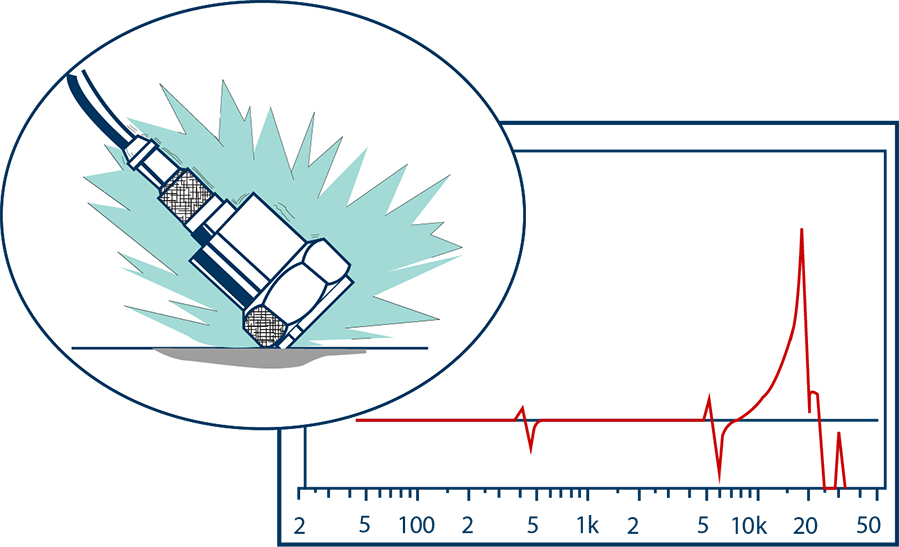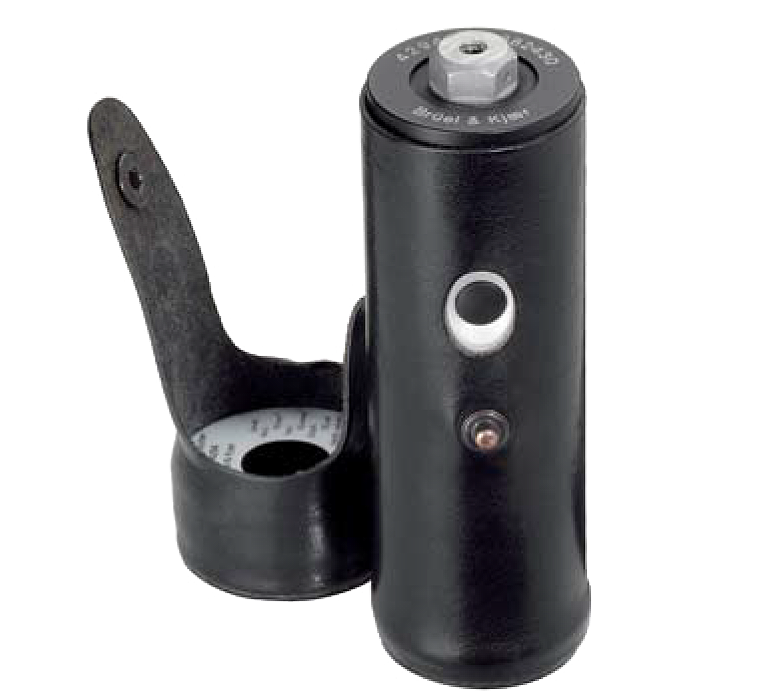## Simple Calibrator for Accelerometers

The most convenient means of performing a periodic calibration check is by using a B&K battery-powered calibrated vibration source. This has a small built-in shaker table which generates precisely 10 m/s2 at 159.15 Hz.

The sensitivity calibration of an accelerometer is checked by fastening it to the shaker table and noting its output when vibrated at 10m/s2.

An equally useful application for the portable calibrator is the checking of a complete measuring or analyzing setup before the measurements are made. The measuring accelerometer is simply transferred from the measuring object to the calibrator and vibrated at a level of 10 m/s2. The readout can be checked and if a recording is being made, the 10 m/s2 calibration level can be recorded for future reference.

## Force and Impedance Measurements

Force transducers are used in mechanical-dynamics measurements together with accelerometers to determine the dynamic forces in a structure and the resulting vibratory motions. The parameters together describe the mechanical impedance of the structure.

The force transducer also uses a piezoelectric element, which when compressed gives an electrical output proportional to the force transmitted through it. The force signals can be processed and measured with the same instrumentation used with accelerometers.

For point impedance measurements on very light structures, the accelerometer and force transducer can be combined into a single unit called an impedance head. Most impedance measurements, however, are performed using a separate accelerometer and force transducer.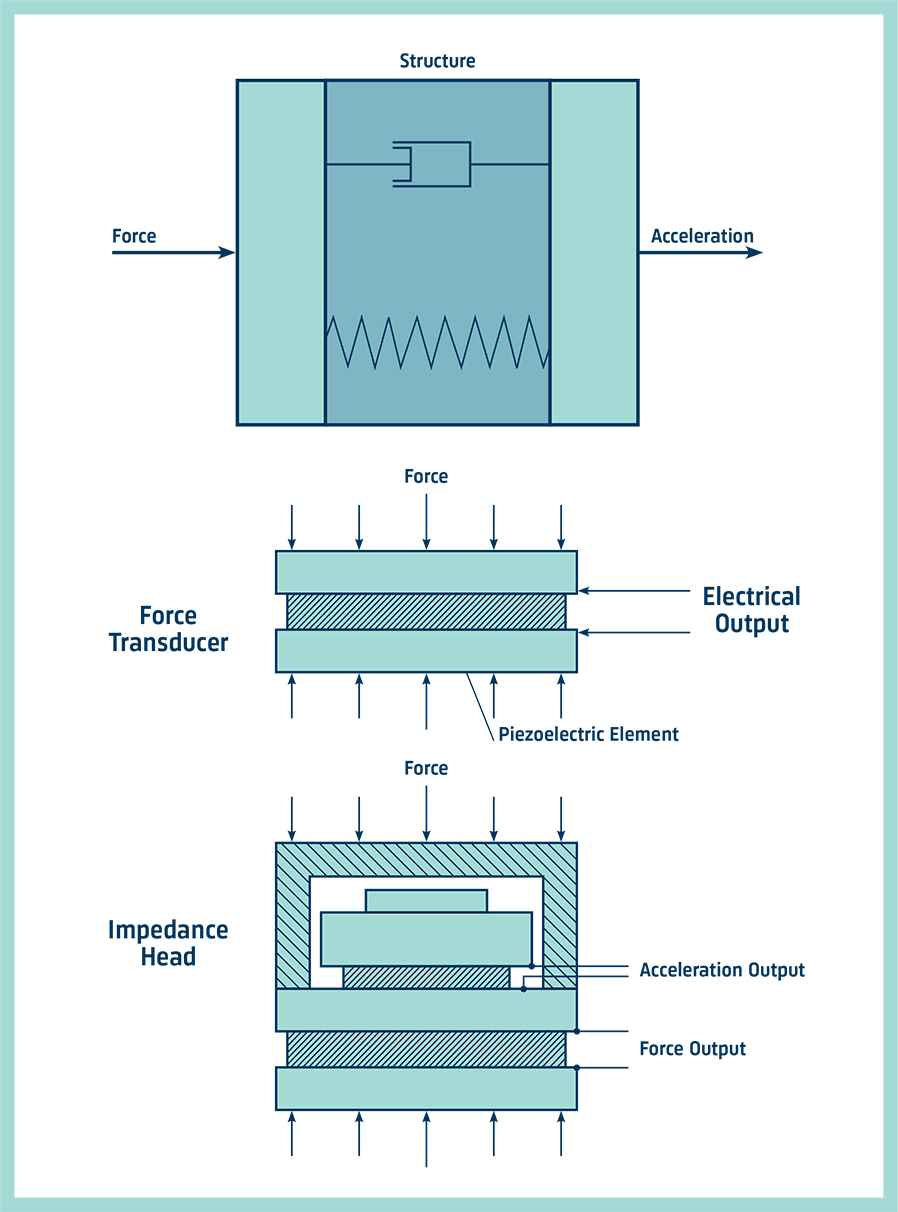## Logarithmic Scales and Decibels

We often plot frequency on a logarithmic scale. This has the effect of expanding the lower frequencies and compressing the higher frequencies on the chart or screen, thus giving the same percentage resolution over the whole width of the chart or screen and keeping its size down to reasonable proportions.

Logarithmic scales are also used to plot vibration amplitudes; this enables the decibel scale to be used as a help in comparing levels. The decibel (dB) is the ratio of one level to another and therefore has no dimensions. But to quote absolute vibration levels, the reference level must be stated.

For example, we can say that one vibration level is 10 dB greater than another level without any further explanation, but if we wish to say that a vibration level is 85 dB, we have to refer it to a reference level. We should say, therefore, that the vibratory acceleration level is 85 dB re. 10-6 m/s2.